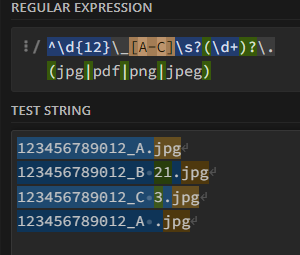# Regex to check if filename was followed

Hi,

I need to check if file name convention was followed with format

12-digitAcctNo_(A|B|C)(.pdf|.jpg)

e.g.

Input: 123456789012_A.jpg
Result: True

Input: 123456789012_B 2.jpg
Result: True

Input: 123456789012_C 3.jpg
Result: True

Input: 123456789012_A .jpg
Result: True

It should allow spaces/characters in any position

I 'm using \b(\d{12})_(A|B|C).(jpg|pdf|png|jpeg) BUT it doesn’t allow space in any position.

Thanks.

@mnlatam

Try below Regular expression.

`\b(\d{12}+)_(A|B|C)..+(jpg|pdf|png|jpeg)`

1 Like

Hi @mnlatam ,

Sorry but its unclear when you said that the space can be anywhere in the string.
If the examples you have provided are examples of where all the spaces can be, then this ought to work out for you →``````^\d{12}\_[A-C]\s?(\d+)?\.(jpg|pdf|png|jpeg)
``````

If you want a space to show up anywhere in between, or if there is a chance of it showing up anywhere in between, then you can simply add this →

``````\s?
``````

This tells the engine that there could be a space at that particular location.

Kind Regards,
Ashwin A.K

1 Like

@mnlatam This expression is working for both the cases

\d{12}(\s+)?_(\s+)?\D(\s+)?.(\s+)?(jpg|pdf|png|jpeg)

Case1 : Data with spaces

Case2: Data without spaces

1 Like